# 22 Pressure Definition; Absolute & Gauge Pressure

Learning Objectives

By the end of this section, you should be able to:

Calculate pressure using its definition

Understand the difference between absolute & gauge pressure

## Physical Properties

Physical properties are properties that can be measured without changing the molecular structure of the substance.

The three main physical properties we will introduce in this lecture are pressure, temperature, and volume:

Pressure – the amount of force exerted per area in a system

Temperature – a measure of the average kinetic energy of a system

Volume – the space occupied by a system

To see more about how these are related for gases, there is an interesting gas property simulator available online: https://phet.colorado.edu/en/simulation/gas-properties

## Pressure $^{}$

When a gas molecule elastically collides with the wall of a container, it exerts a force on the wall. These forces are the source of pressure in a gas. In a sample of gas in a container, the randomness of the molecular motion causes the number of collisions to fluctuate in a given time. However, because a huge number of molecules collide with the wall in a short time, the number of collisions on the scales of time and space we measure fluctuates by only a tiny, usually unobservable fraction from the average.

As the number of molecules increases, the number of collisions, and thus the pressure, increases. If the average velocity of the molecules is higher, each collision exerts a larger force on the wall, therefore the gas pressure is higher.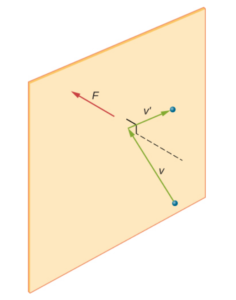Image obtained from OpenStax University Physics Volume 2 / CC BY 4.0

### Calculation and Units:

$Pressure (P)=\frac{force}{area}$

$\text{units for } P= \frac{ML}{t^2}L^{-2}=\frac{M}{LT^2}$

General notation:

M – any units of mass (g, kg, lbs…)

L – any units of length (m, cm, in…)

t – any units of time (s, min, hr…)

Common units for pressure:

[S.I.] $\frac{kg}{ms^2}=\frac{N}{m^2}=Pa$ (i.e. Pascal)

[cgs] $\frac{g}{cms^2} = \frac{dyne}{cm^2}$

[American] $\frac{lbm}{fts^2}$

Other common pressure units:

$1k\!Pa (kilo-Pascal) = 10^3 Pa$

$1M\!Pa (mega-Pascal) = 10^6 Pa$

$1bar= 10^5 Pa = 10^5 N/m^2$

$1 atm = 1.013×10^5 Pa = 760 mmHg$ (millimetres of mercury column at 0 °C) = $14.7 psi$ (i.e. $lbf/in^2$ pound force per square inch)

Exercise: Pressure Calculation

What is the force exerted from a column of air on us in mass per square metre?

Take atmosphere pressure = $1.013×10^5 Pa$

### Solution

We can use Newton’s second law $F=ma$ to find mass, with $a$ being the acceleration of gravity. Since the mass we are looking for is “per square metre”, we can also divide the force by area(in $m^2$), which gives us pressure on the left side of the equation.

$mass\;(per\;m^2)=\frac{P}{a}=\frac{1.013×10^5Pa}{9.81\frac{m}{s^2}}=\frac{1.013×10^5\frac{kg}{ms^2}}{9.81\frac{m}{s^2}}=10326\frac{kg}{m^2}$

## Measuring Pressure (Manometers) $^{}$

A manometer can be used to determine gas pressures. The manometer is essentially a U-shaped tube containing some kind of fluid with known density, and one side is connected to the region of interest while the reference pressure is applied to the other. The difference in liquid level represents the applied pressure.

Manometer fluid – mercury was used originally (hence mmHg), but we now have a wide variety of fluids of various densities.

Manometer types:

1. open-ended manometer:

$P_{ref} = P_{atm}$

1. sealed-end manometer:

$P_{ref} = \text{whatever it is set to (generally ~0, vacuum)}$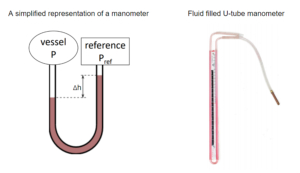Right side image obtained from Apply Science Concepts to Trades Applications / CC BY 4.0

The difference in heights of the liquid columns correlates to the difference in the pressure of the gases. The pressure of the gas in the vessel is calculated by:

$P=P_{ref}+\rho g(h_{ref}-h)$

Exercise: Manometer Calculation

Compressed air has been used to transmit and store power since the 1870s. Cities such as Paris, Dresden and Buenos Aires had compressed air lines to transmit power to homes and businesses. The technology is now being applied to energy storage in former salt mines underground. One such plant in McIntosh, Alabama stores gas at 1100 psi. If a sealed end manometer with a fluid with a density of 3450 $\frac{𝑘𝑔}{𝑚^3}$ was used to measure this pressure, what would the height of the fluid column be? (take $g=9.8m/s^2$)

### Solution

Manipulate h from the equation:

$P=P_{ref}+\rho g(h_{ref}-h)$

\begin{align*}
h& = \frac{P-P_{0}}{\rho g}\\
h& = \frac{1100psi×\frac{101325Pa}{14.696psi}}{3450\frac{kg}{m^3}×9.8\frac{m}{s^2}}\\
&=\frac{7.58×10^6Pa}{3.381×10^4\frac{kg}{m^2s^2}} \\
& = 2.242×10^2 \frac{Pa}{\frac{kg}{m^2s^2}}×\frac{1\frac{kg}{ms^2}}{1Pa}\\
& = 2.24×10^2m
\end{align*}

height = $2.2410^2m$ or 224 m, very high pressure, likely difficult to measure with a manometer.

Gauge vs. Absolute Pressure

The absolute pressure is the actual pressure at the point of interest. The absolute pressure is 0 in a vacuum and cannot be negative.

Gauge pressure is defined to be the difference between absolute pressure and atmospheric pressure:

$gauge\; pressure\; =\; absolute\; pressure\; – \;atmospheric\; pressure$

when gauge pressure is 0, absolute pressure = atmospheric pressure

Most measuring devices measure gauge pressure. Many measuring devices use a flexible membrane between a chamber of known pressure (the outside chamber, which is connected to the atmosphere) and a vessel with the pressure we want to measure. If there is a difference in pressure on both sides of the membrane, the membrane will expand or contract. Through this, we can relate the membrane’s position to the gauge pressure of the vessel.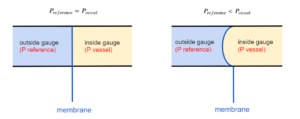The units often used on the devices include:

$psi$ = pounds per square inch

$inH\!g$ = inches of mercury

Exercise: Reading Absolute and Gauge Pressures

1. If the gauge in the image reads 5 psi at the orange mark and the atmospheric pressure is 14.7 psi, which of the following are the gauge and absolute pressures?

A – gauge = -5 psi, absolute = 9.7 psi

B – gauge = 5 psi, absolute = 9.7 psi

C – gauge = -5 psi, absolute = 19.7 psi

D – gauge = 5 psi, absolute = 19.7 psi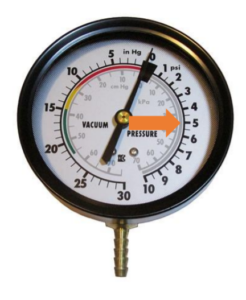2. If the gauge in the image reads 10 inHg at the purple mark and the atmospheric pressure is 29.9 inHg, which of the following are the gauge and absolute pressures?

A – gauge = -10 inHg, absolute = 39.9 inHg

B – gauge = 10 inHg, absolute = 19.9 inHg

C – gauge = -10 inHg, absolute = 19.9 inHg

D – gauge = 10 inHg, absolute = 39.9 inHg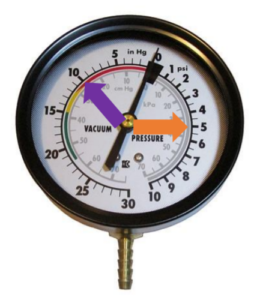### Solution

The arrow is on the “pressure” side, which indicates that the gauge pressure is positive. The absolute pressure is the sum of absolute pressure and gauge pressure.
\begin{align*}
absolute\;pressure& =gauge\;pressure+atmospheric\;pressure\\
& = 5psi+14.7psi\\
&=19.7 psi
\end{align*}

The arrow is on the “vacuum” side, which indicates that the gauge pressure is negative. The absolute pressure is the sum of absolute pressure and gauge pressure.
\begin{align*}
absolute\;pressure& =gauge\;pressure+atmospheric\;pressure\\
& =-10inH\!g+29.9inH\!g\\
&=19.9inH\!g
\end{align*}

## References

 OpenStax University Physics Volume 2. 2016. 2.2 Pressure, Temperature, and RMS Speed. [online]<https://openstax.org/books/university-physics-volume-2/pages/2-2-pressure-temperature-and-rms-speed> [Accessed 11 May 2020].

 Line D – Organizational Skills Competency D-2: Apply Science Concepts to Trades Applications. 2015. Manometer. [online] <http://solr.bccampus.ca:8001/bcc/file/3654f43b-8e2b-43be-99ce-acd1b2e4d809/1/D-2.pdf> [Accessed 11 May 2020].